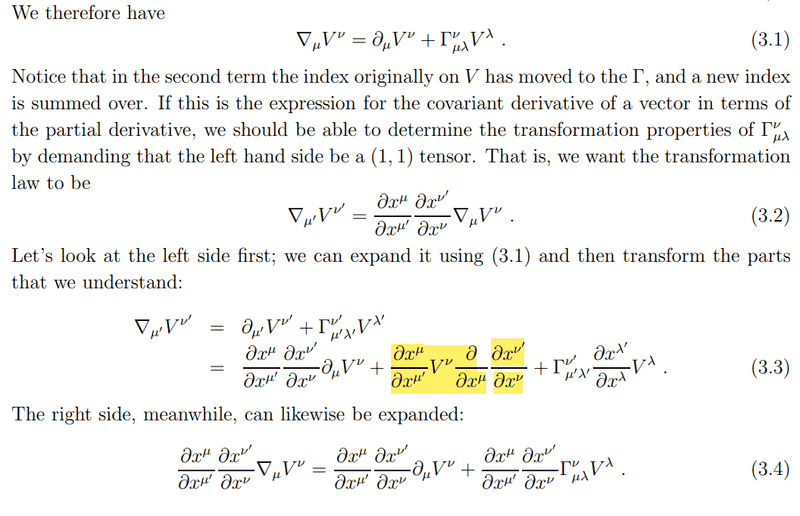# Transformation of connection coefficients

• I
• accdd

#### accdd

I don't understand why the highlighted term is there.
This image was taken from Sean Carroll's notes available here: preposterousuniverse.com/wp-content/uploads/grnotes-three.pdfThis follows directly (together with the first term) from writing out the transformation relation ##V^{\nu’} = \frac{\partial x^{\nu’}}{\partial x^\nu} V^\nu## and applying the Leibniz rule.

•accdd
Thanks for the answer, could you show me the steps to get to the result?

I think it would be more instructive if you do what I proposed starting from the first line in (3.3) and show us the steps until you get stuck.

•strangerep and accdd
$$V^{\nu'}=\frac{\partial x^{\nu'}}{\partial x^{\nu}}V^{\nu}$$
$$\nabla_{\mu'}V^{\nu'}=\partial_{\mu'}(\frac{\partial x^{\nu'}}{\partial x^{\nu}}V^{\nu})+\Gamma^{\nu'}_{\mu'\lambda'}(\frac{\partial x^{\lambda'}}{\partial x^{\lambda}}V^{\lambda}) =\partial_{\mu'}(\frac{\partial x^{\nu'}}{\partial x^{\nu}})V^{\nu}+\frac{\partial x^{\nu'}}{\partial x^{\nu}} \partial_{\mu'}V^{\nu}+\Gamma^{\nu'}_{\mu'\lambda'}\frac{\partial x^{\lambda'}}{\partial x^{\lambda}}V^{\lambda}=$$

Ok. So the piece that you're missing is expressing ##\partial_{\mu'}## in terms of ##\partial_\mu##. Are you familiar with any way to relate those two partial derivatives?

•accdd
Is this the correct transformation?
$$\partial_{\mu'}=\frac{\partial x^\mu}{\partial x^{\mu'}}\partial_\mu$$
$$\partial_{\mu'}(\frac{\partial x^{\nu'}}{\partial x^{\nu}})V^{\nu}+\frac{\partial x^{\nu'}}{\partial x^{\nu}} \partial_{\mu'}V^{\nu}+\Gamma^{\nu'}_{\mu'\lambda'}\frac{\partial x^{\lambda'}}{\partial x^{\lambda}}V^{\lambda}= \frac{\partial x^\mu}{\partial x^{\mu'}}\partial_\mu(\frac{\partial x^{\nu'}}{\partial x^{\nu}})V^{\nu}+\frac{\partial x^{\nu'}}{\partial x^{\nu}} \frac{\partial x^\mu}{\partial x^{\mu'}}\partial_\mu V^{\nu}+\Gamma^{\nu'}_{\mu'\lambda'}\frac{\partial x^{\lambda'}}{\partial x^{\lambda}}V^{\lambda}$$

so the first line of 3.3 should be more clearly:
$$\nabla_{\mu'}V^{\nu'}=(\frac{\partial x^\mu}{\partial x^{\mu'}}\partial_\mu)(\frac{\partial x^{\nu'}}{\partial x^{\nu}}V^{\nu})+\Gamma^{\nu'}_{\mu'\lambda'}(\frac{\partial x^{\lambda'}}{\partial x^{\lambda}}V^{\lambda})$$

Last edited:
Is this the correct transformation?
$$\partial_{\mu'}=\frac{\partial x^\mu}{\partial x^{\mu'}}\partial_\mu$$
Yes. That is the chain rule for partial derivatives.

•accdd